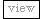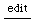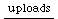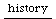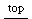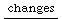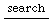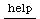## a simple markov set ugen graph

from: http://www.create.ucsb.edu/pipermail/sc-users/2004-October/013674.html (idea by Tom Gersic)

```(
SynthDef("markov", { arg w1=#[0, 0, 1], w2=#[1,0,0], w3=#[0,1,0];
var trig, index, derPitch, previousIndex;

trig = Impulse.kr(6);
previousIndex = LocalIn.kr(1); // access the last cycle's index output
derPitch=[w1, w2, w3];
derPitch = derPitch.flop; // you want an array of Select ugens here, each one switching a row.
// so when previousIndex is 0, the three selects have the values of the first set of weights.
// then as a next index 2 results, which switches the weight selects to weights 2

index = TWindex.kr(trig, Select.kr(previousIndex, derPitch), 1); // auto normalize

LocalOut.kr(index); // make the new index available to the next cycle

Out.ar(0,
SinOsc.ar(
Select.kr(index,[400, 500, 600]),
0, 0.2
)
)
}).send(s);
)
a = Synth(\markov);

a.setn(\w1, [1, 0, 1]);
a.setn(\w2, [0, 1, 1]);
```